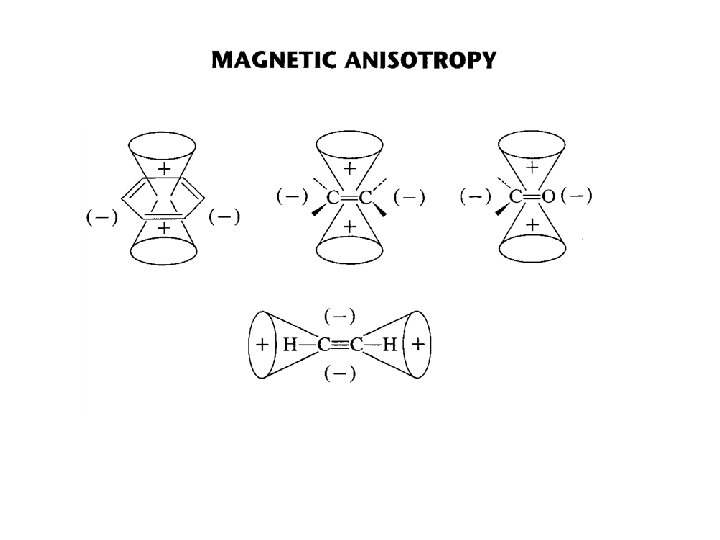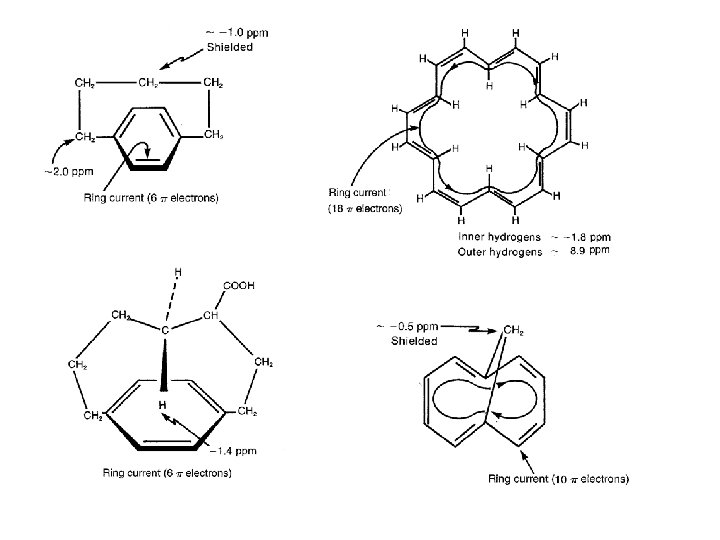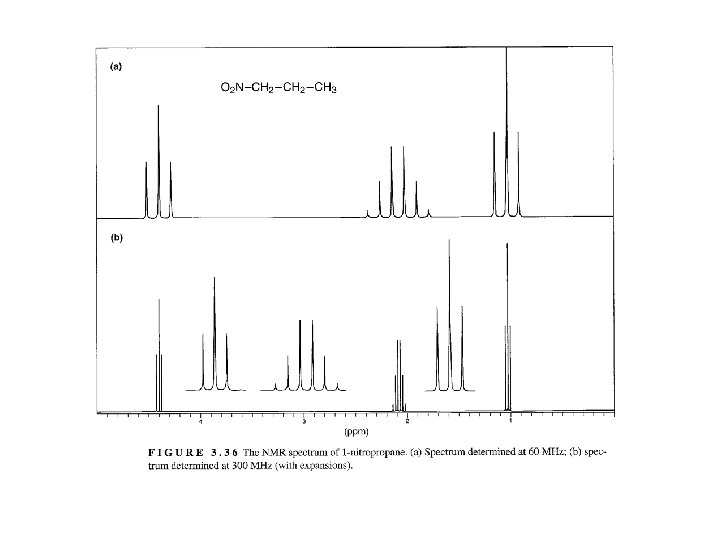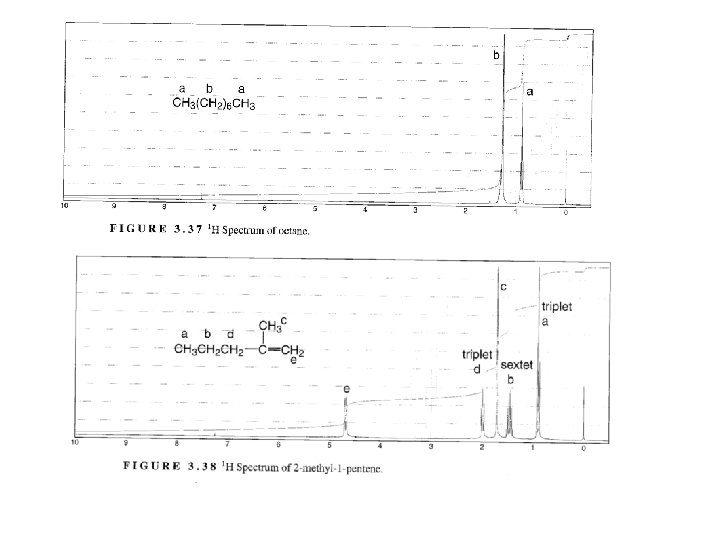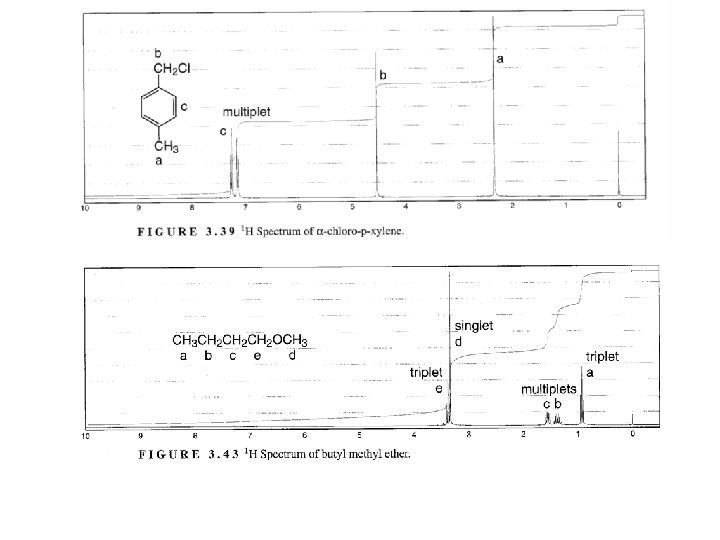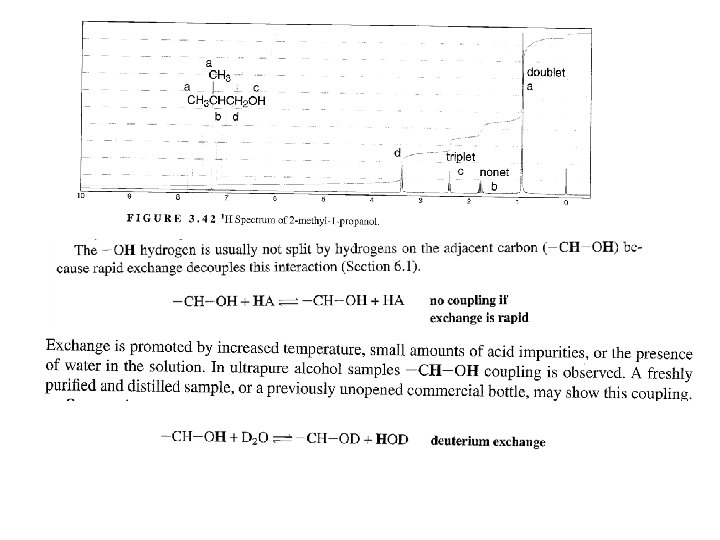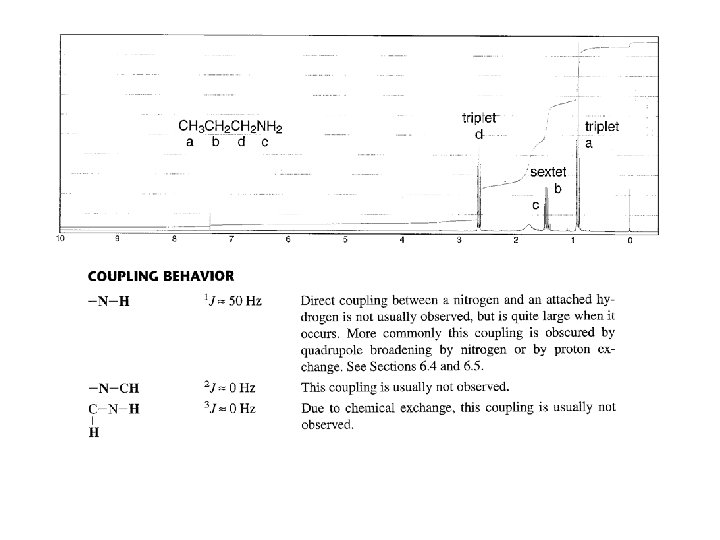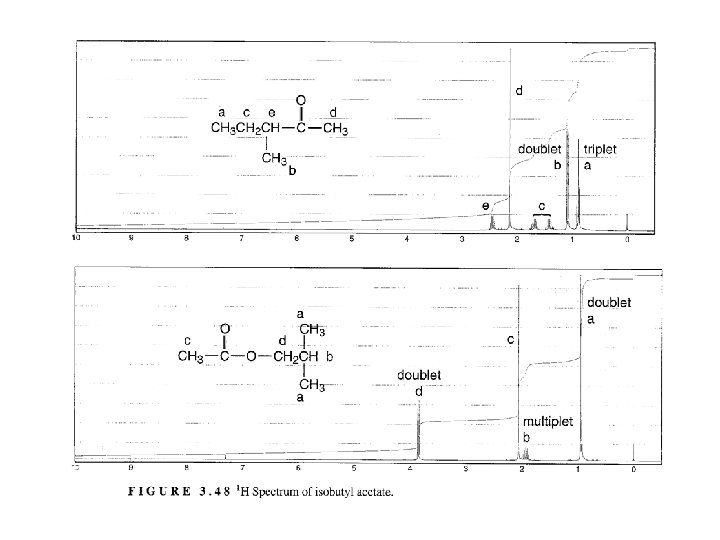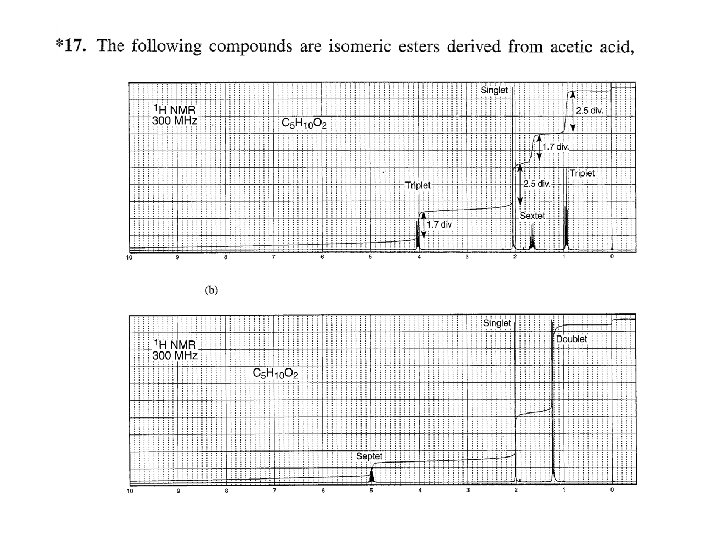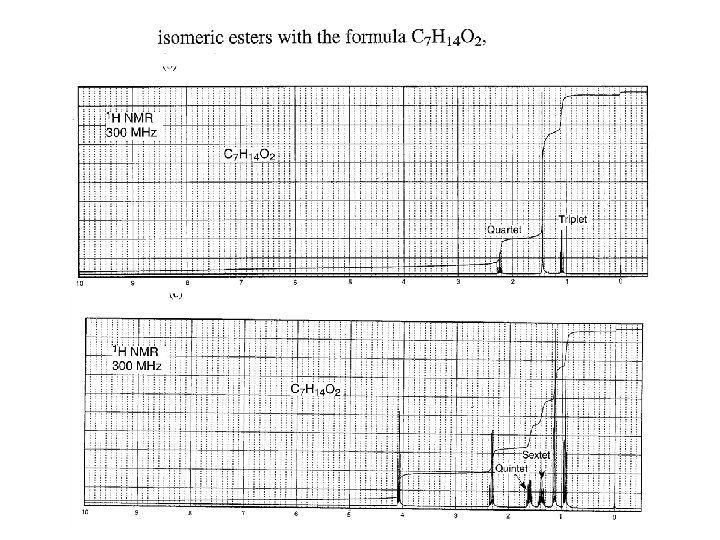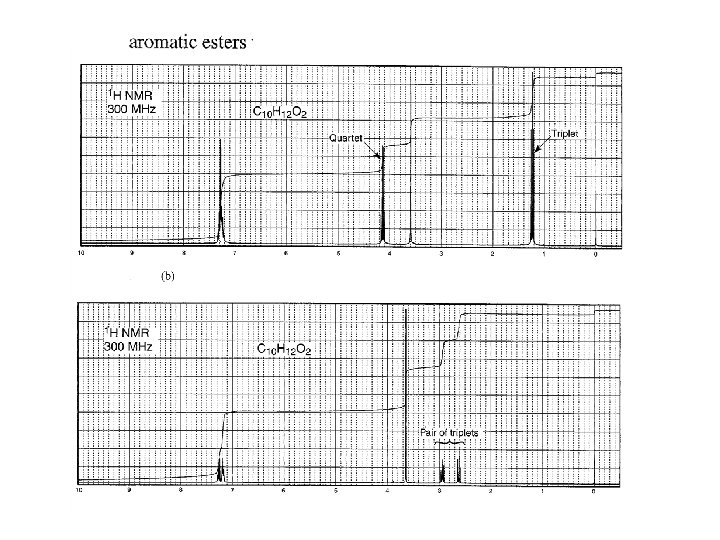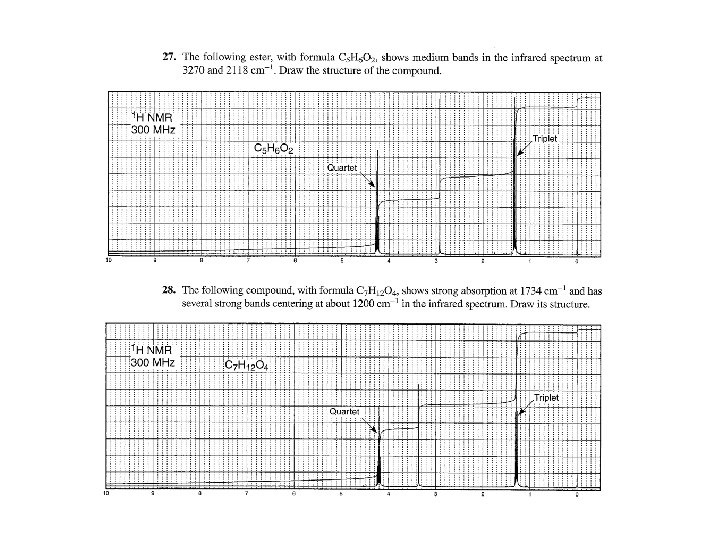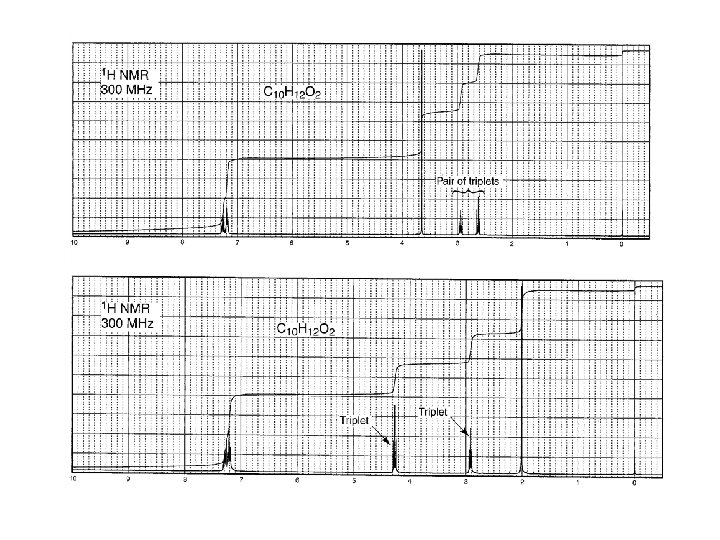Скачать презентацию SPIN-SPIN SPLITTING SPIN-SPIN SPLITTING Often a group

5ecf8c8ec4f9031b7b5c31acc1b6d673.ppt

• Количество слайдов: 47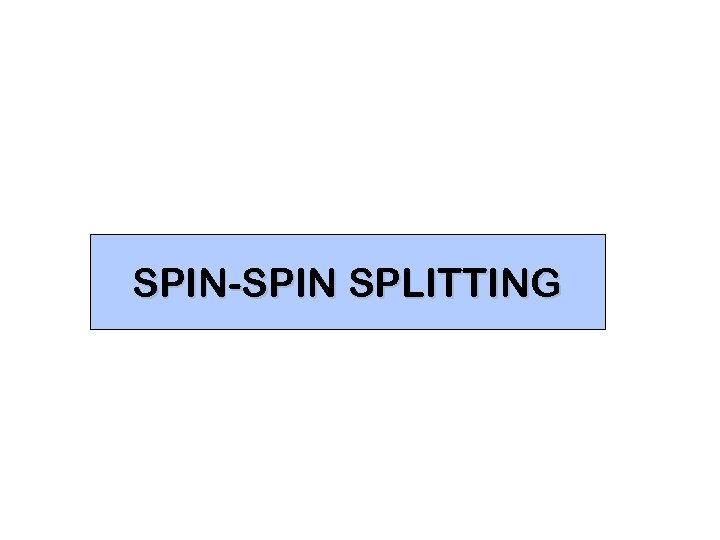SPIN-SPIN SPLITTING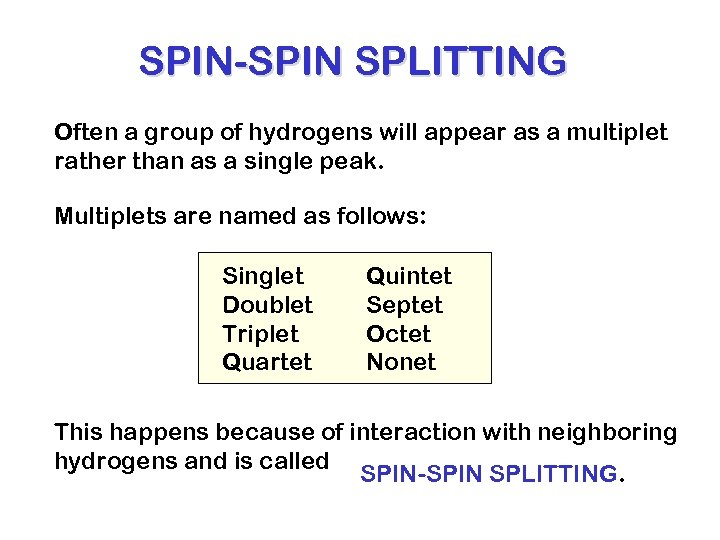SPIN-SPIN SPLITTING Often a group of hydrogens will appear as a multiplet rather than as a single peak. Multiplets are named as follows: Singlet Doublet Triplet Quartet Quintet Septet Octet Nonet This happens because of interaction with neighboring hydrogens and is called SPIN-SPIN SPLITTING.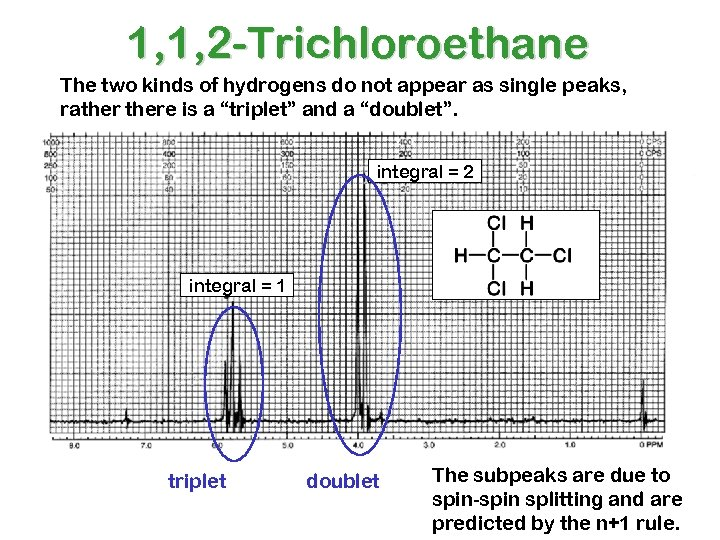1, 1, 2 -Trichloroethane The two kinds of hydrogens do not appear as single peaks, rathere is a “triplet” and a “doublet”. integral = 2 integral = 1 triplet doublet The subpeaks are due to spin-spin splitting and are predicted by the n+1 rule.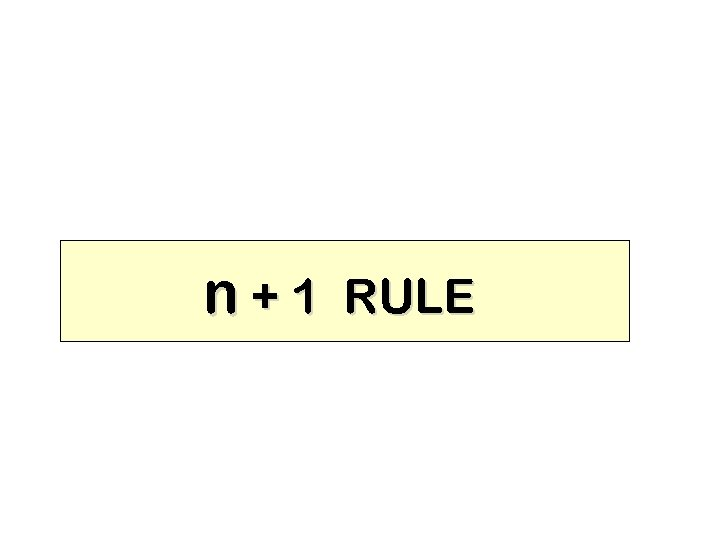n+1 RULE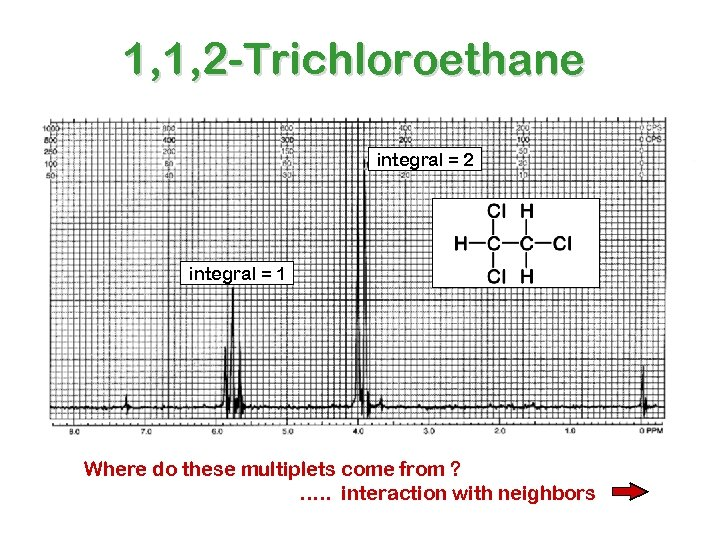1, 1, 2 -Trichloroethane integral = 2 integral = 1 Where do these multiplets come from ? …. . interaction with neighbors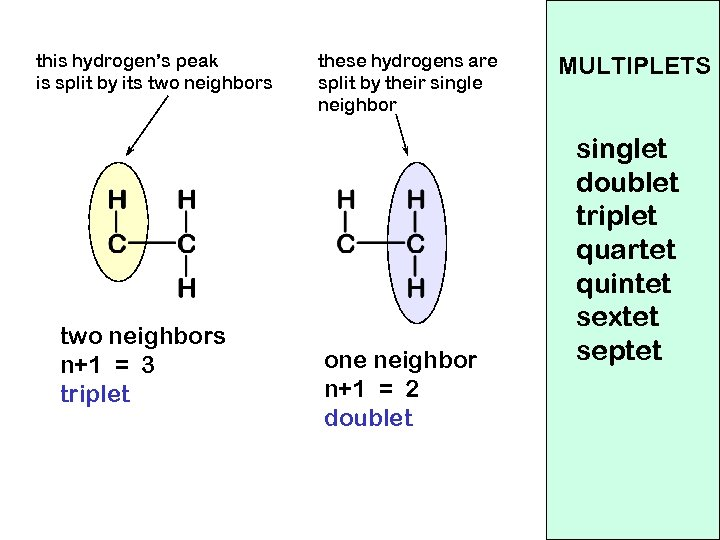this hydrogen’s peak is split by its two neighbors n+1 = 3 triplet these hydrogens are split by their single neighbor one neighbor n+1 = 2 doublet MULTIPLETS singlet doublet triplet quartet quintet sextet septet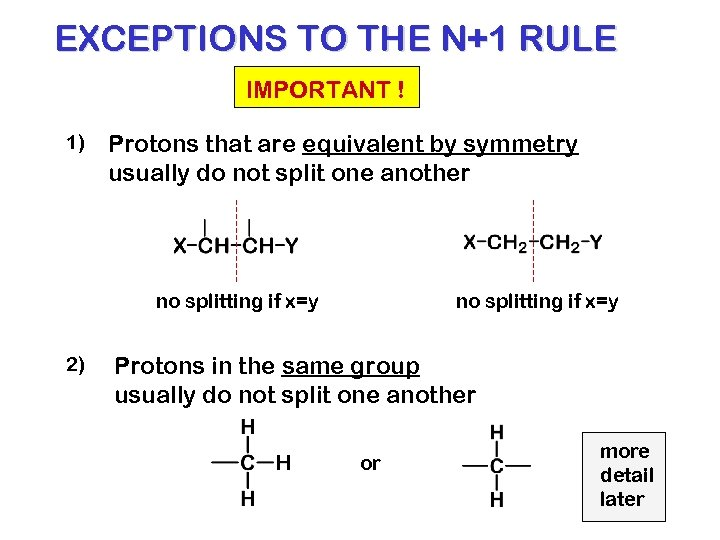EXCEPTIONS TO THE N+1 RULE IMPORTANT ! 1) Protons that are equivalent by symmetry usually do not split one another no splitting if x=y 2) no splitting if x=y Protons in the same group usually do not split one another or more detail later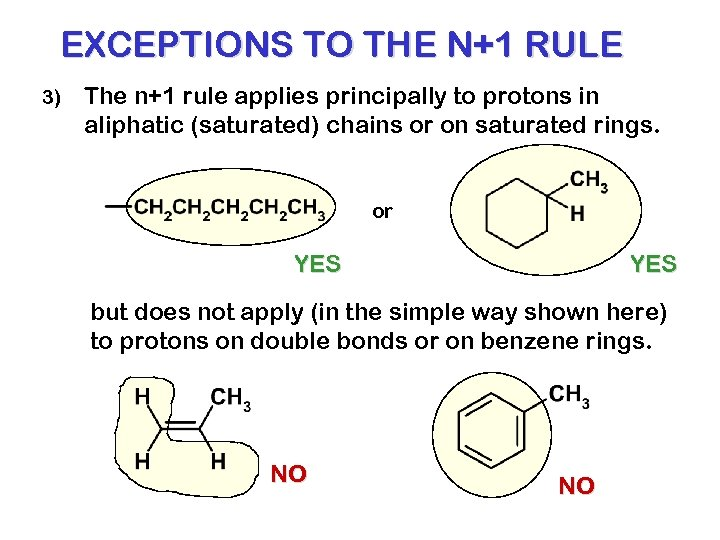EXCEPTIONS TO THE N+1 RULE 3) The n+1 rule applies principally to protons in aliphatic (saturated) chains or on saturated rings. or YES but does not apply (in the simple way shown here) to protons on double bonds or on benzene rings. NO NO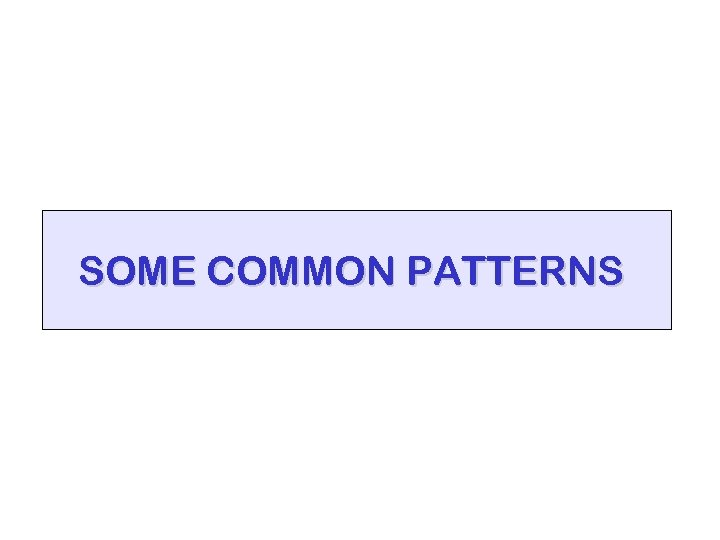SOME COMMON PATTERNS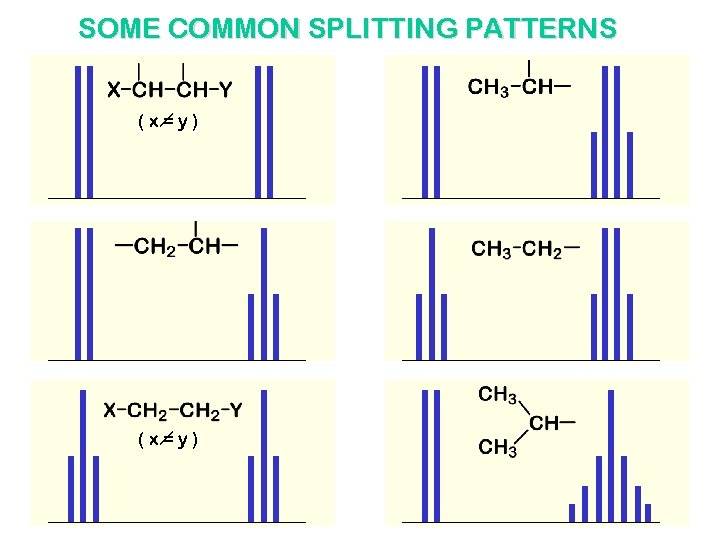SOME COMMON SPLITTING PATTERNS (x=y)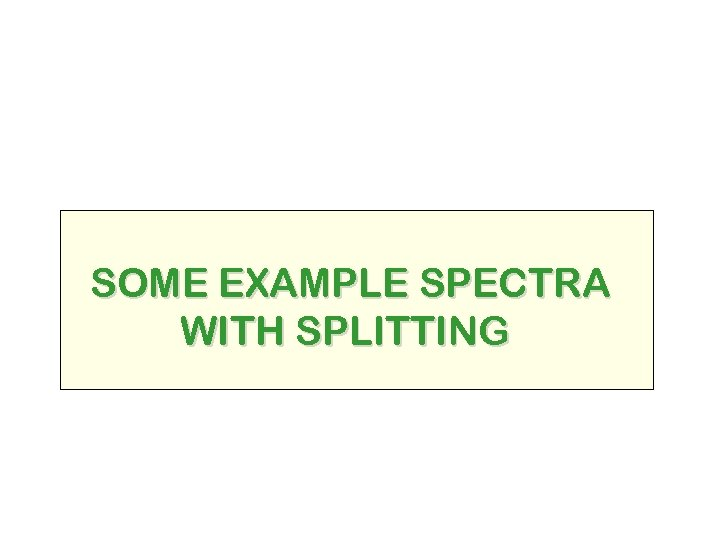SOME EXAMPLE SPECTRA WITH SPLITTING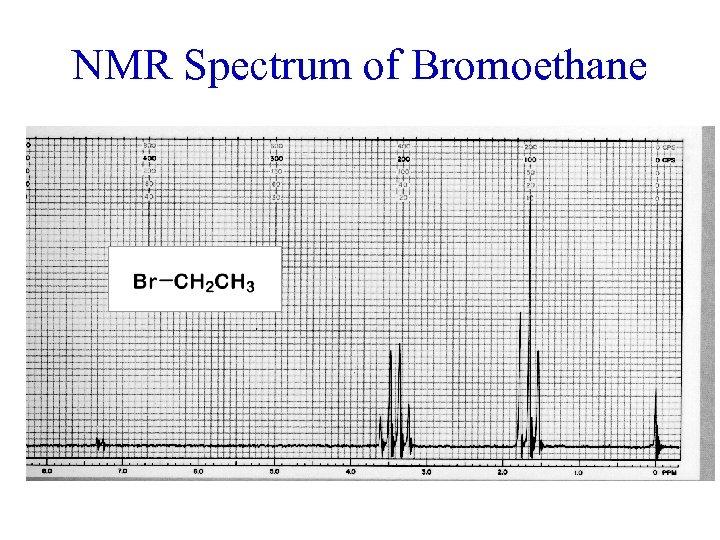NMR Spectrum of Bromoethane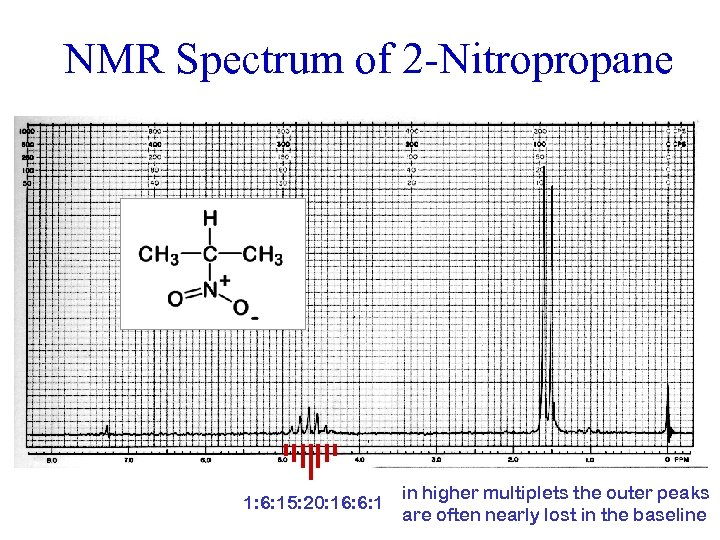NMR Spectrum of 2 -Nitropropane 1: 6: 15: 20: 16: 6: 1 in higher multiplets the outer peaks are often nearly lost in the baseline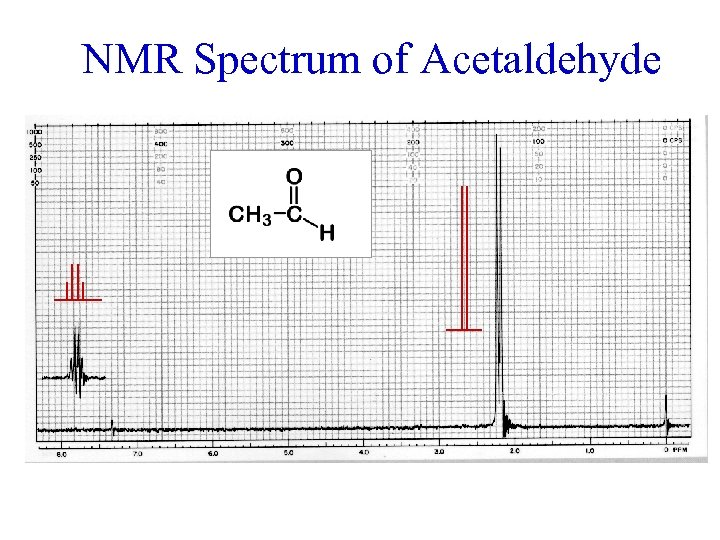NMR Spectrum of Acetaldehyde offset = 2. 0 ppm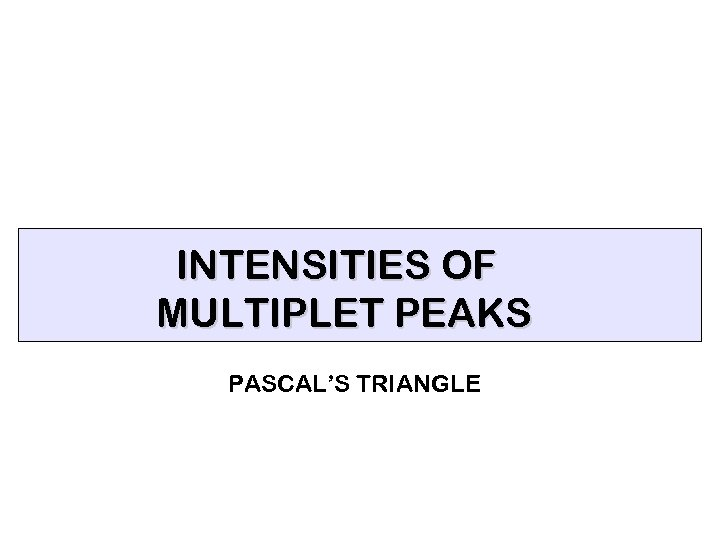INTENSITIES OF MULTIPLET PEAKS PASCAL’S TRIANGLE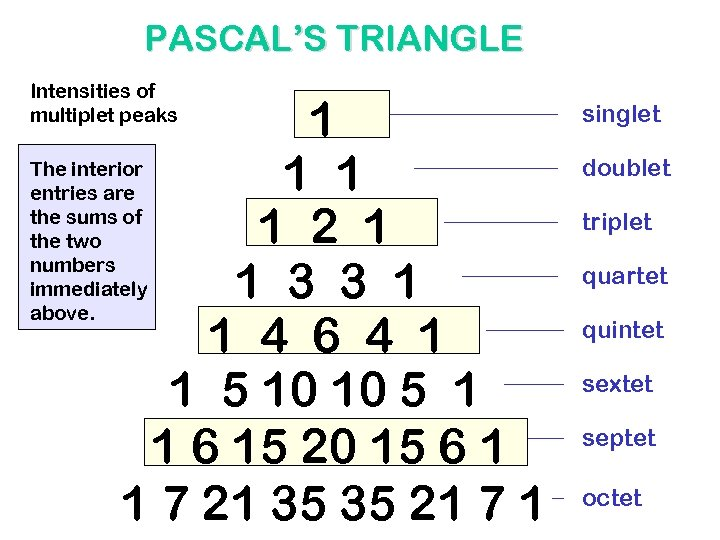PASCAL’S TRIANGLE Intensities of multiplet peaks 1 The interior 1 1 entries are the sums of 1 2 1 the two numbers immediately 1 3 3 1 above. 1 4 6 4 1 1 5 10 10 5 1 1 6 15 20 15 6 1 1 7 21 35 35 21 7 1 singlet doublet triplet quartet quintet sextet septet octet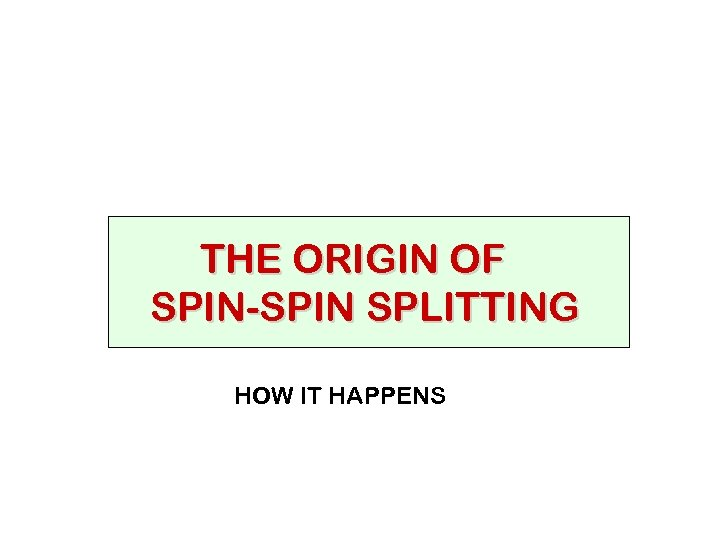THE ORIGIN OF SPIN-SPIN SPLITTING HOW IT HAPPENS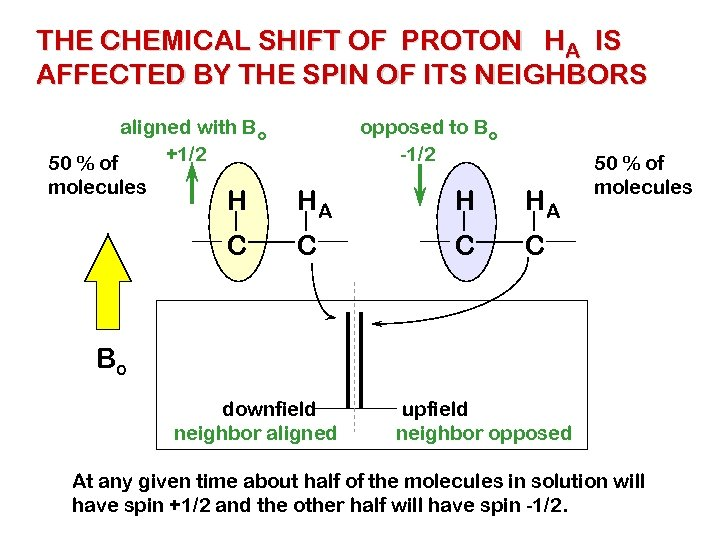THE CHEMICAL SHIFT OF PROTON HA IS AFFECTED BY THE SPIN OF ITS NEIGHBORS aligned with Bo +1/2 50 % of molecules opposed to Bo -1/2 H HA C C C 50 % of molecules C Bo downfield neighbor aligned upfield neighbor opposed At any given time about half of the molecules in solution will have spin +1/2 and the other half will have spin -1/2.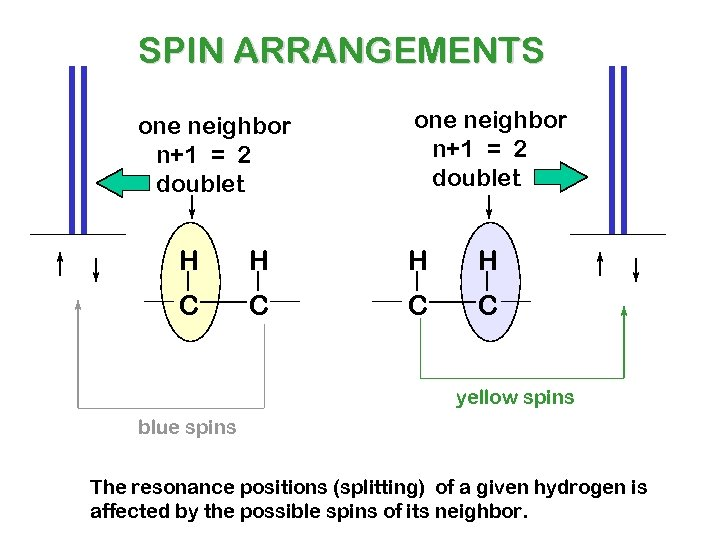SPIN ARRANGEMENTS one neighbor n+1 = 2 doublet H H C C yellow spins blue spins The resonance positions (splitting) of a given hydrogen is affected by the possible spins of its neighbor.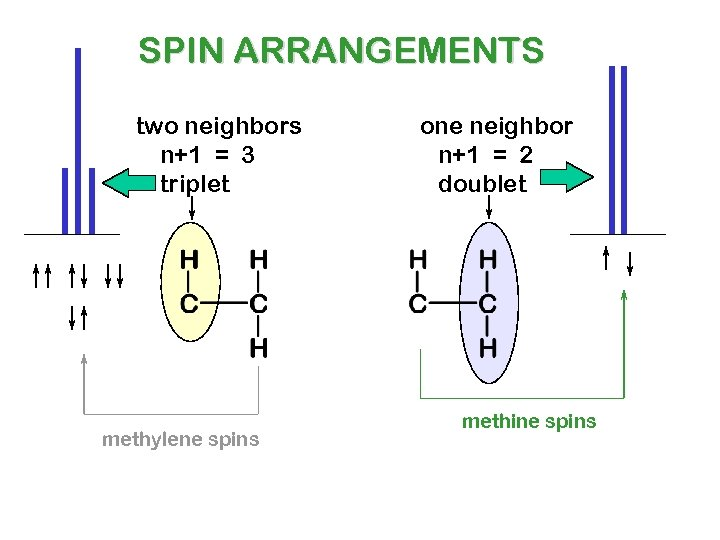SPIN ARRANGEMENTS two neighbors n+1 = 3 triplet methylene spins one neighbor n+1 = 2 doublet methine spins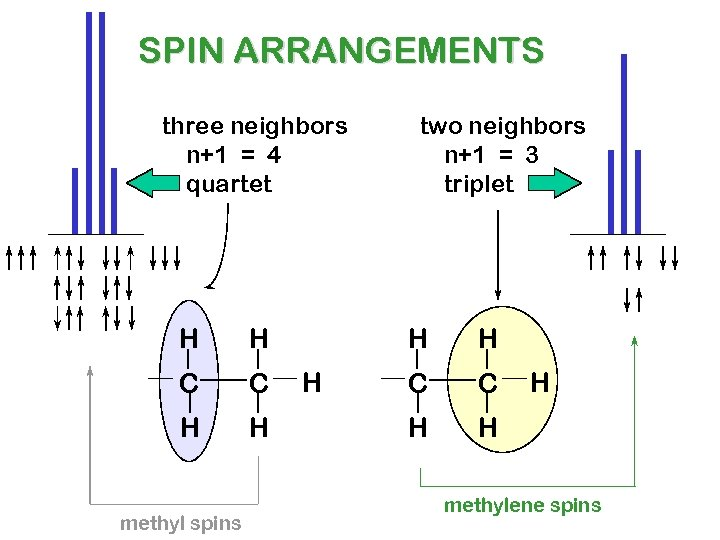SPIN ARRANGEMENTS three neighbors n+1 = 4 quartet H H C C H H methyl spins two neighbors n+1 = 3 triplet H H H C C H H H methylene spins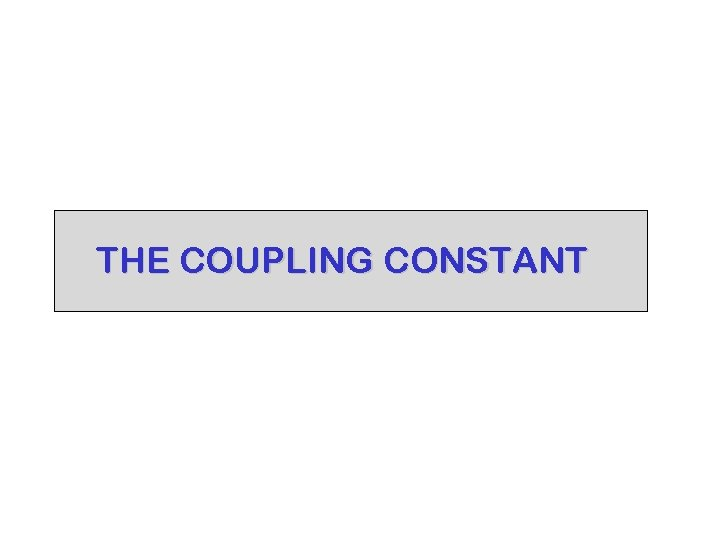THE COUPLING CONSTANT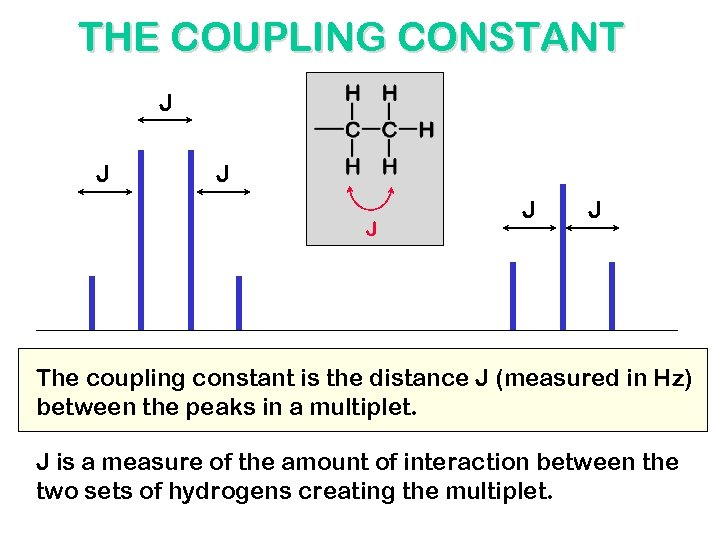THE COUPLING CONSTANT J J J The coupling constant is the distance J (measured in Hz) between the peaks in a multiplet. J is a measure of the amount of interaction between the two sets of hydrogens creating the multiplet.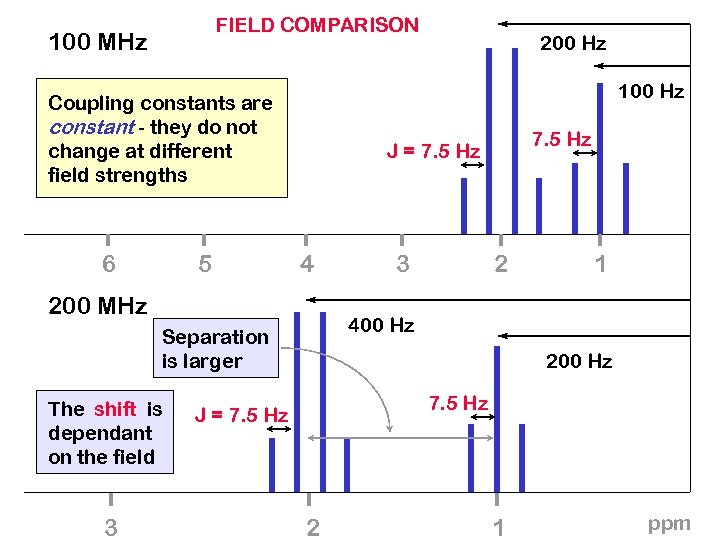FIELD COMPARISON 100 MHz 100 Hz Coupling constants are constant - they do not change at different field strengths 6 5 4 3 2 1 400 Hz Separation is larger 3 7. 5 Hz J = 7. 5 Hz 200 MHz The shift is dependant on the field 200 Hz 7. 5 Hz J = 7. 5 Hz 2 1 ppm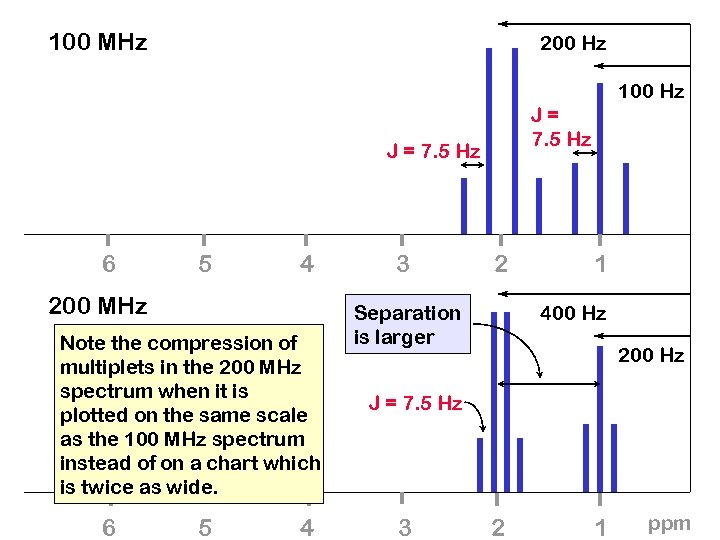100 MHz 200 Hz 100 Hz J= 7. 5 Hz J = 7. 5 Hz 6 5 4 200 MHz Note the compression of multiplets in the 200 MHz spectrum when it is plotted on the same scale as the 100 MHz spectrum instead of on a chart which is twice as wide. 6 5 4 3 2 Separation is larger 1 400 Hz 200 Hz J = 7. 5 Hz 3 2 1 ppm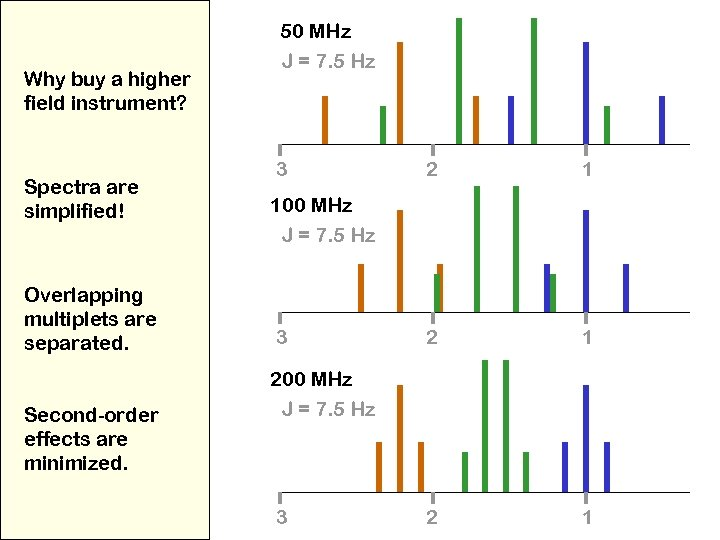Why buy a higher field instrument? Spectra are simplified! Overlapping multiplets are separated. Second-order effects are minimized. 50 MHz J = 7. 5 Hz 3 2 1 2 1 100 MHz J = 7. 5 Hz 3 200 MHz J = 7. 5 Hz 3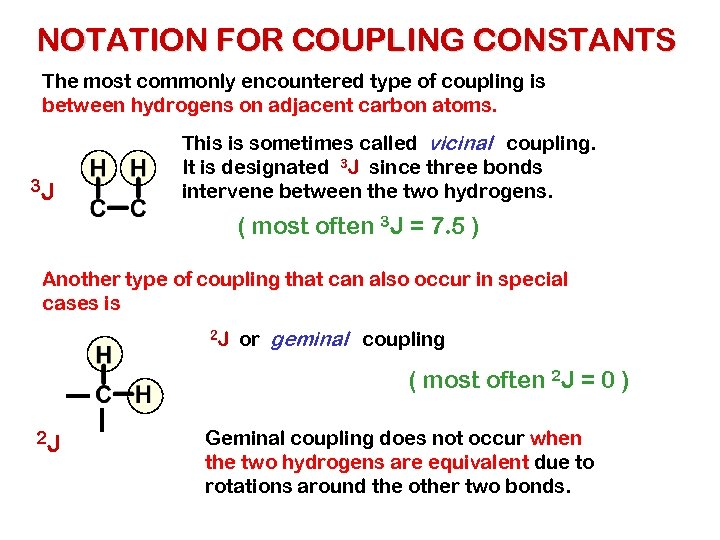NOTATION FOR COUPLING CONSTANTS The most commonly encountered type of coupling is between hydrogens on adjacent carbon atoms. 3 J This is sometimes called vicinal coupling. It is designated 3 J since three bonds intervene between the two hydrogens. ( most often 3 J = 7. 5 ) Another type of coupling that can also occur in special cases is 2 J or geminal coupling ( most often 2 J = 0 ) 2 J Geminal coupling does not occur when the two hydrogens are equivalent due to rotations around the other two bonds.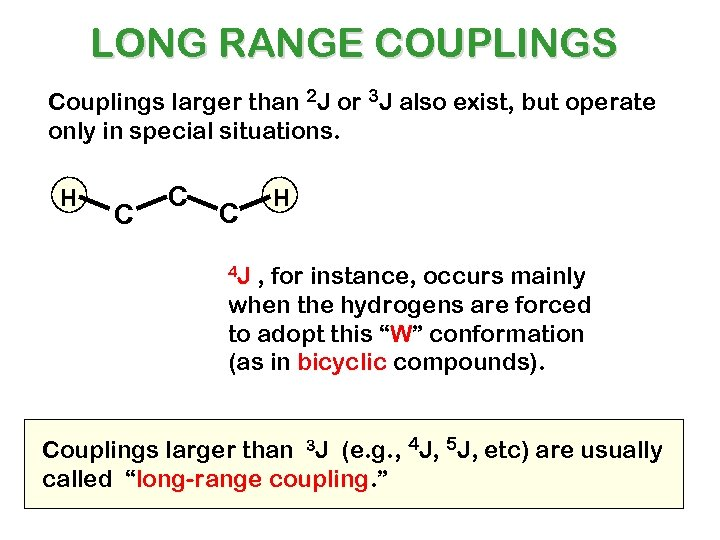LONG RANGE COUPLINGS Couplings larger than 2 J or 3 J also exist, but operate only in special situations. H C C C H 4 J , for instance, occurs mainly when the hydrogens are forced to adopt this “W” conformation (as in bicyclic compounds). Couplings larger than 3 J (e. g. , 4 J, 5 J, etc) are usually called “long-range coupling. ”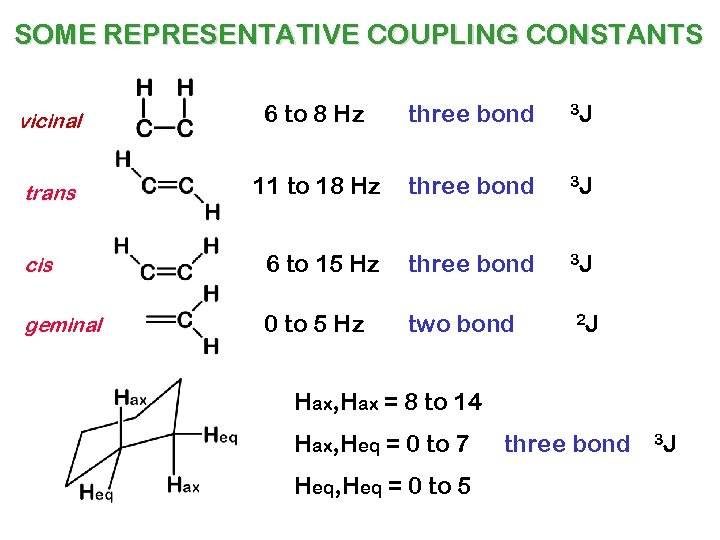SOME REPRESENTATIVE COUPLING CONSTANTS vicinal 6 to 8 Hz three bond 3 J trans 11 to 18 Hz three bond 3 J cis 6 to 15 Hz three bond 3 J geminal 0 to 5 Hz two bond 2 J Hax, Hax = 8 to 14 Hax, Heq = 0 to 7 Heq, Heq = 0 to 5 three bond 3 J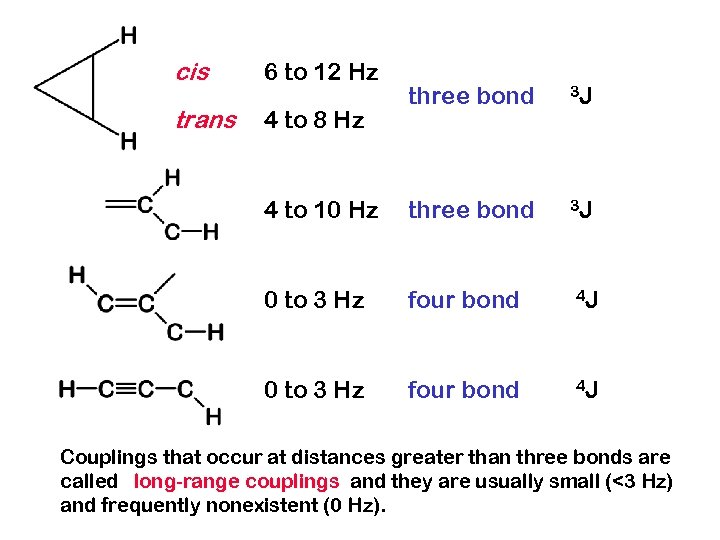cis 6 to 12 Hz trans 4 to 8 Hz three bond 3 J 4 to 10 Hz three bond 3 J 0 to 3 Hz four bond 4 J Couplings that occur at distances greater than three bonds are called long-range couplings and they are usually small (<3 Hz) and frequently nonexistent (0 Hz).OVERVIEW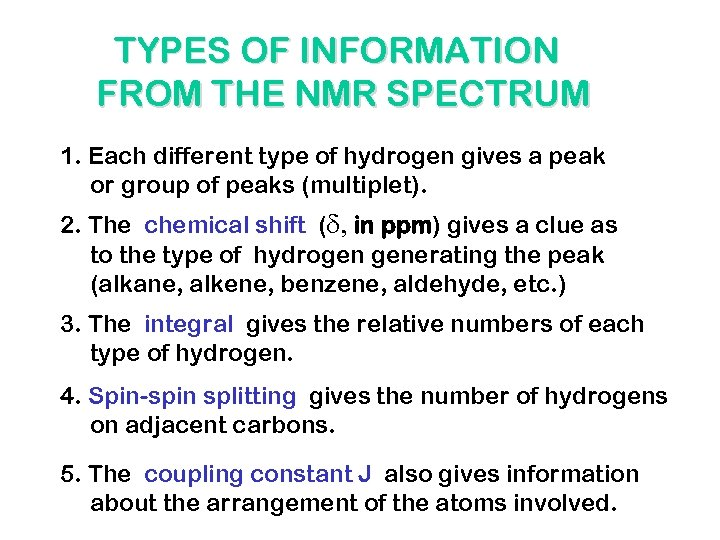TYPES OF INFORMATION FROM THE NMR SPECTRUM 1. Each different type of hydrogen gives a peak or group of peaks (multiplet). 2. The chemical shift (d, in ppm) gives a clue as to the type of hydrogen generating the peak (alkane, alkene, benzene, aldehyde, etc. ) 3. The integral gives the relative numbers of each type of hydrogen. 4. Spin-spin splitting gives the number of hydrogens on adjacent carbons. 5. The coupling constant J also gives information about the arrangement of the atoms involved.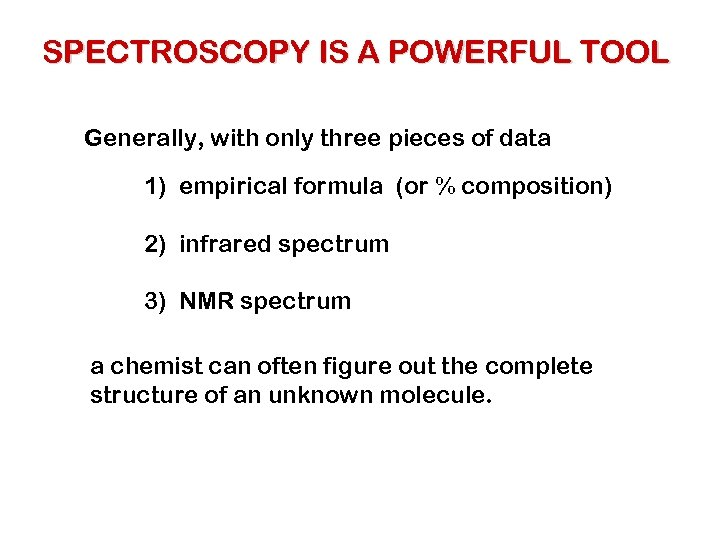SPECTROSCOPY IS A POWERFUL TOOL Generally, with only three pieces of data 1) empirical formula (or % composition) 2) infrared spectrum 3) NMR spectrum a chemist can often figure out the complete structure of an unknown molecule.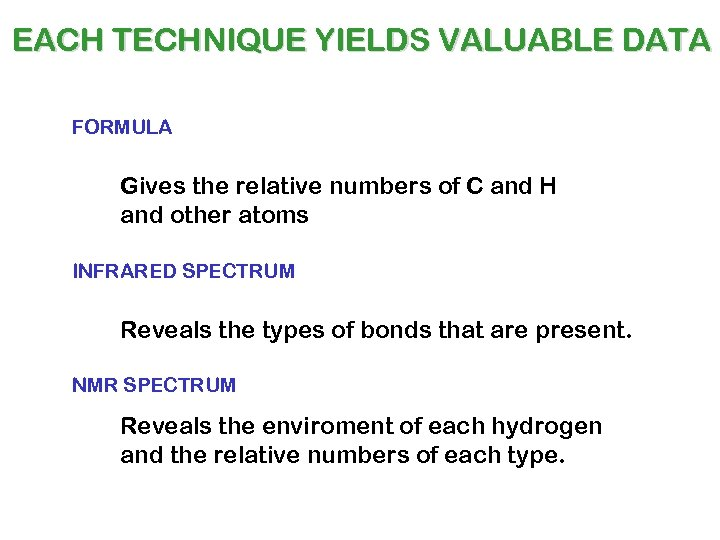EACH TECHNIQUE YIELDS VALUABLE DATA FORMULA Gives the relative numbers of C and H and other atoms INFRARED SPECTRUM Reveals the types of bonds that are present. NMR SPECTRUM Reveals the enviroment of each hydrogen and the relative numbers of each type.